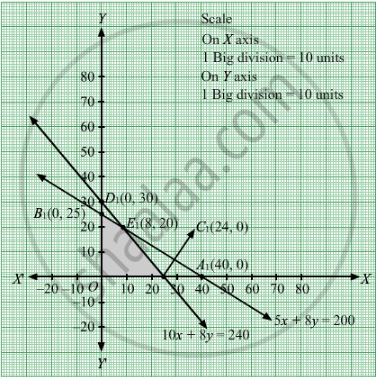# A Company Manufactures Two Types of Novelty Souvenirs Made of Plywood. Souvenirs of Type a Require 5 Minutes Each for Cutting and 10 Minutes Each for Assembling . - Mathematics

Sum

A company manufactures two types of novelty Souvenirs made of plywood. Souvenirs of type A require 5 minutes each for cutting and 10 minutes each for assembling. Souvenirs of type B require 8 minutes each for cutting and 8 minutes each for assembling. There are 3 hours 20 minutes available for cutting and 4 hours available for assembling. The profit is 50 paise each for type A and 60 paise each for type B souvenirs. How many souvenirs of each type should the company manufacture in order to maximize the profit?

#### Solution

Let the company manufacture x souvenirs of type A and y souvenirs of type B.
Number of items cannot be negative.
Therefore,
≥ 0 and ≥ 0

The given information can be complied in a table as follows.

 Type A Type B Availability cutting (min) 5 8 3x60+20=200 Assembling (min) 10 8 4 x 60 = 240

Therefore, the constraints are

5x + 8y ≤ 200
10x + 8y ≤ 240

The profit on type A souvenirs is 50 paise and on type B souvenirs is 60 paise.Therefore, profit gained on x souvenirs of type A and y souvenirs of type is Rs 0.50x and Rs 0.60y respectively.

Total profit, Z = 0.5x + 0.6y

The mathematical formulation of the given problem is

Maximize Z = 0.5x + 0.6y

subject to the constraints,

5x + 8y ≤ 200
10x + 8y ≤ 240
≥ 0 and ≥ 0

First we will convert inequations into equations as follows:
5x + 8y = 200, 10x + 8y = 240, x = 0 and y = 0
Region represented by 5x + 8y ≤ 200:
The line 5x + 8y = 200 meets the coordinate axes at A1(40, 0) and B1(0, 25) respectively. By joining these points we obtain the line 5x + 8y = 200 . Clearly (0,0) satisfies the 5x + 8y = 200. So,
the region which contains the origin represents the solution set of the inequation 5x + 8y ≤ 200.

Region represented by 10x + 8y ≤ 240:
The line 10x + 8y = 240 meets the coordinate axes at C1(24, 0) and D1(0, 30) respectively. By joining these points we obtain the line 10x + 8y = 240. Clearly (0,0) satisfies the inequation 10x + 8y ≤ 240. So,
the region which contains the origin represents the solution set of the inequation 10x + 8y ≤ 240.

Region represented by ≥ 0 and y ≥ 0:
Since, every point in the first quadrant satisfies these inequations. So, the first quadrant is the region represented by the inequations x ≥ 0, and y ≥ 0.
The feasible region determined by the system of constraints 5x + 8y ≤ 200, 10x + 8y ≤ 240, x ≥ 0 and y ≥ 0 are as follows.The corner points of the feasible region are O(0, 0), B1(0, 25), E1(8, 20), C1(24, 0).

The values of Z at these corner points are as follows.

 Corner point Z = 0.5x + 0.6y O(0, 0) 0 B1(0, 25) 15 E1(8, 20) 16 C1(24, 0) 12

The maximum value of Z is 160 at E1(8, 20).Thus, 8 souvenirs of type A and 20 souvenirs of type B should be produced each day to get the maximum profit of Rs 16.

Concept: Graphical Method of Solving Linear Programming Problems
Is there an error in this question or solution?

#### APPEARS IN

RD Sharma Class 12 Maths
Chapter 30 Linear programming
Exercise 30.4 | Q 18 | Page 52